# How do you write brackets[ ] and parenthesis( ) in LaTeX?

Brackets and parenthesis are always needed when representing a complex mathematical expression.

There are many types of brackets and each has a different latex syntax to define. Take a look at this table and code below for example.

LaTeX syntax Output
( abc ) ( abc )
[ abc ] [ abc ]
\{ abc \} { abc }
\langle abc \rangle 〈 abc 〉
\documentclass{article}
\begin{document}
$(abc)$
$[abc]$
$\{xyz\}$
$\langle xyz \rangle$
\end{document}

Output :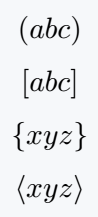First of all, you will want to use the curly braces in direct code from the keyboard like square bracket, but as a result, you will not get any output.

In both right and left cases, you have to use backslash.

\documentclass{article}
\begin{document}
${abc}$
${a_1b_1c_1}$
$\{abc\}$
$\{a_1b_1c_1\}$
\end{document}


Output :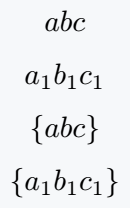Second, your keyboard does not have any angle brackets. But, looking at your less than and greater than symbols, it will look like an angels bracket which is not exactly correct. For this, there is a special command in latex.

\documentclass{article}
\begin{document}
$<\frac{x}{y}>$
$\langle xyz \rangle$
$\left\langle \frac{x}{y}\right\rangle$
\end{document}


Output :## Adjustable size of brackets in LaTeX

Even after knowing the command in the case of each bracket, you will have a problem with a large expression. Because brackets and large expressions will not adjust dynamically.

For this, you need to use \left and \right commands.

\documentclass{article}
\begin{document}
$\left( \frac{f(x)}{k} \right)$
$\left[ \frac{f'(x)}{k} \right]$
$\left\{ \frac{g(x)}{k} \right\}$
$\left\langle \frac{g'(x)}{k} \right\rangle$
\end{document}

Output :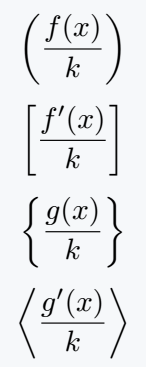## Manually resize brackets and parenthesis

At above point, the expression and size of brackets are being dynamically adjustable.

But, if you want, you can manually adjust with a large expression. For this, you need to know the use of four types of big commands.

\documentclass{article}
\begin{document}
$\Bigg(\bigg(\Big(\big( a \big)\Big)\bigg)\Bigg)$
$\Bigg[\bigg[\Big[\big[ b \big]\Big]\bigg]\Bigg]$
$\Bigg\{\bigg\{\Big\{\big\{ c \big\}\Big\}\bigg\}\Bigg\}$
$\Bigg\langle\bigg\langle\Big\langle\big\langle d \big\rangle\Big\rangle\bigg\rangle\Bigg\rangle$
\end{document}

Output :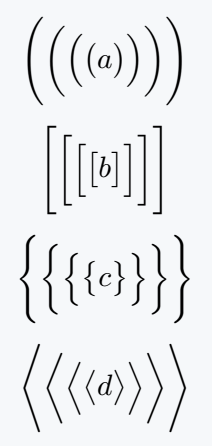## Use physics package for Big Brackets

Physics package will return automatically an adjustable bracket. In this case, you do not need to use \left and \right commands separately.
All you have to do is use the brackets or parenthesis with the \qty command.

\documentclass{article}
\usepackage{physics}
\begin{document}
$\qty(a)\; \qty(\frac{a}{k}) \; \qty(\frac{a_p}{k_n})$
$\qty[b]\; \qty[\frac{b}{k}] \; \qty[\frac{b_p}{k_n}]$
$\qty{a}\; \qty{\frac{a}{k}} \; \qty{\frac{a_p}{k_n}}$
\end{document}

Output :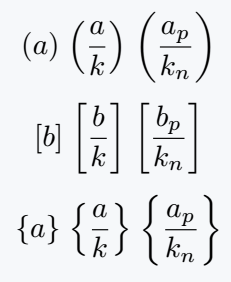There are other ways you can solve this problem. In this case, a special command is defined which is equivalent to the previous command.

\documentclass{article}
\usepackage{physics}
\begin{document}
$\pqty{\frac{f(x)}{g(x)}}$
$\bqty{\frac{f'(x)}{g'(x)}}$
$\bqty{\frac{f''(x)}{g''(x)}}$
\end{document}

Output :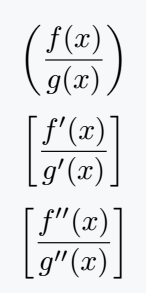You can also manually resize each bracket of physics package. However, after \qty command, four types of big commands have to be used.

\documentclass{article}
\usepackage{physics}
\begin{document}
$\qty\Bigg(\qty\bigg(\qty\Big(\qty\big(L^AT_EX))))$
$\qty\Bigg[\qty\bigg[\qty\Big[\qty\big[Physics]]]]$
$\qty\Bigg{\qty\bigg{\qty\Big{\qty\big{Package}}}}$
\end{document}

Output :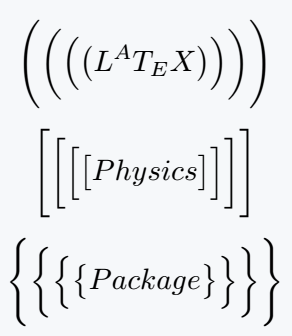#### Md Jidan Mondal

LaTeX expert with over 10 years of experience in document preparation and typesetting. Specializes in creating professional documents, reports, and presentations using LaTeX.